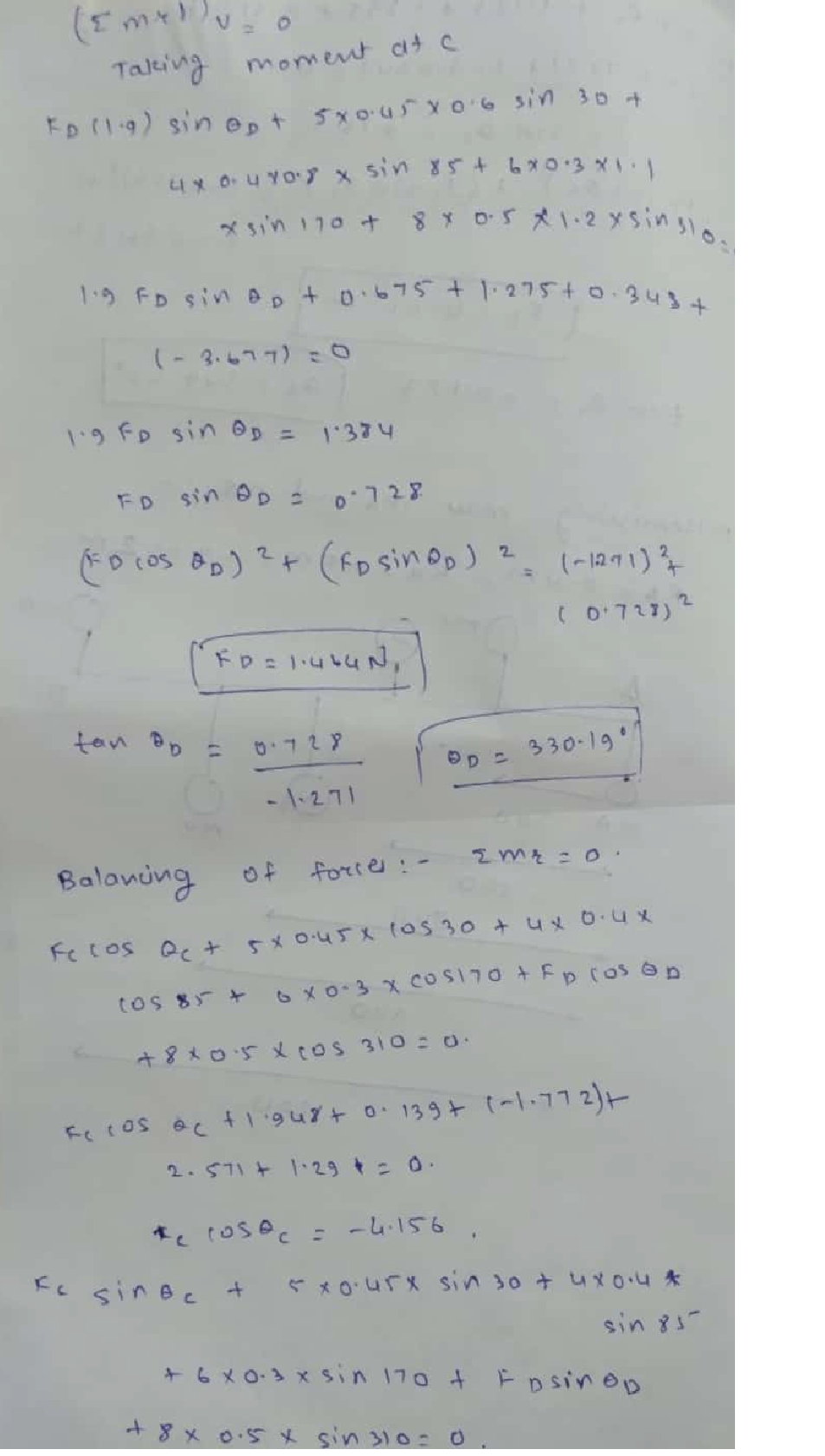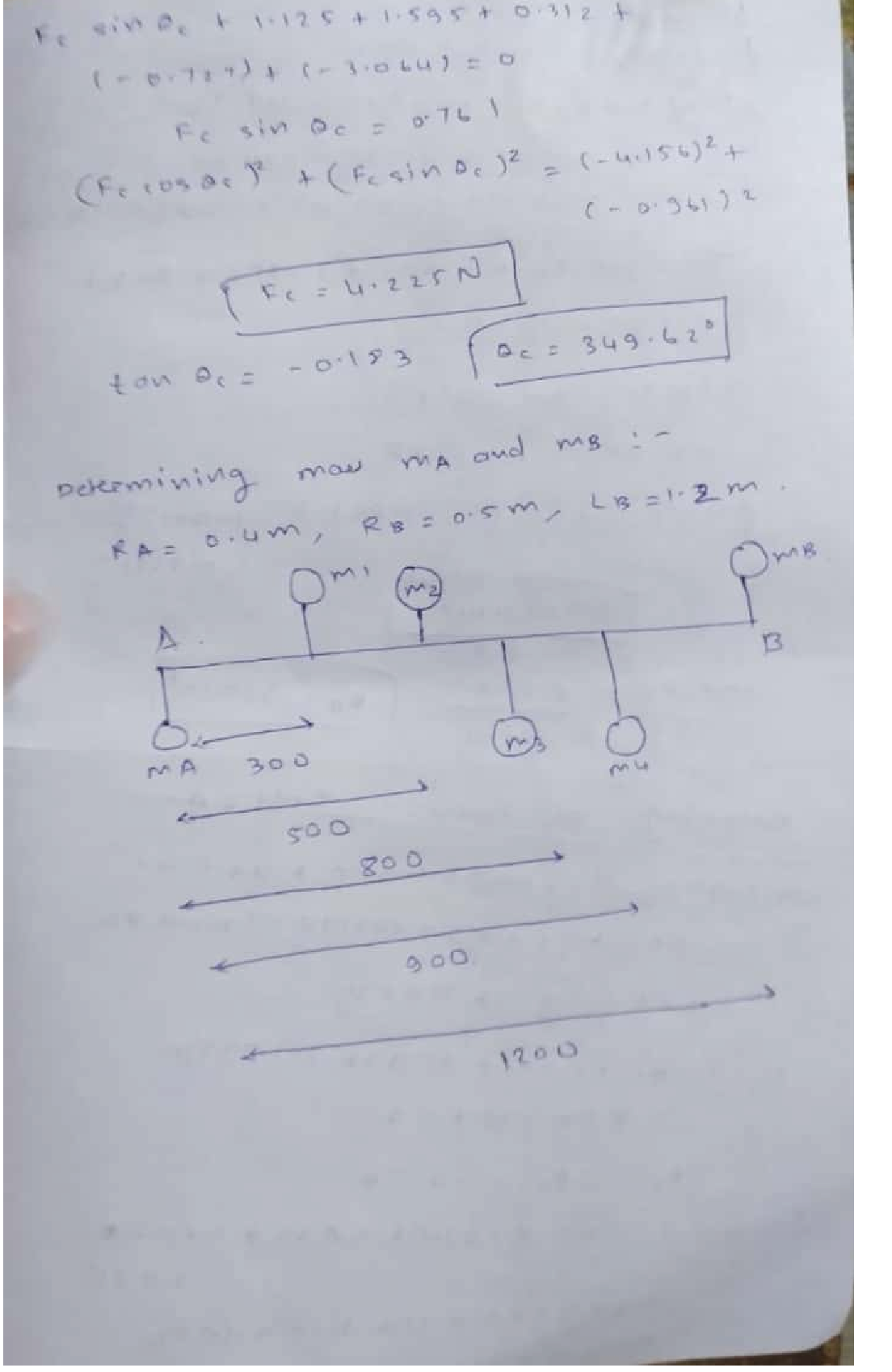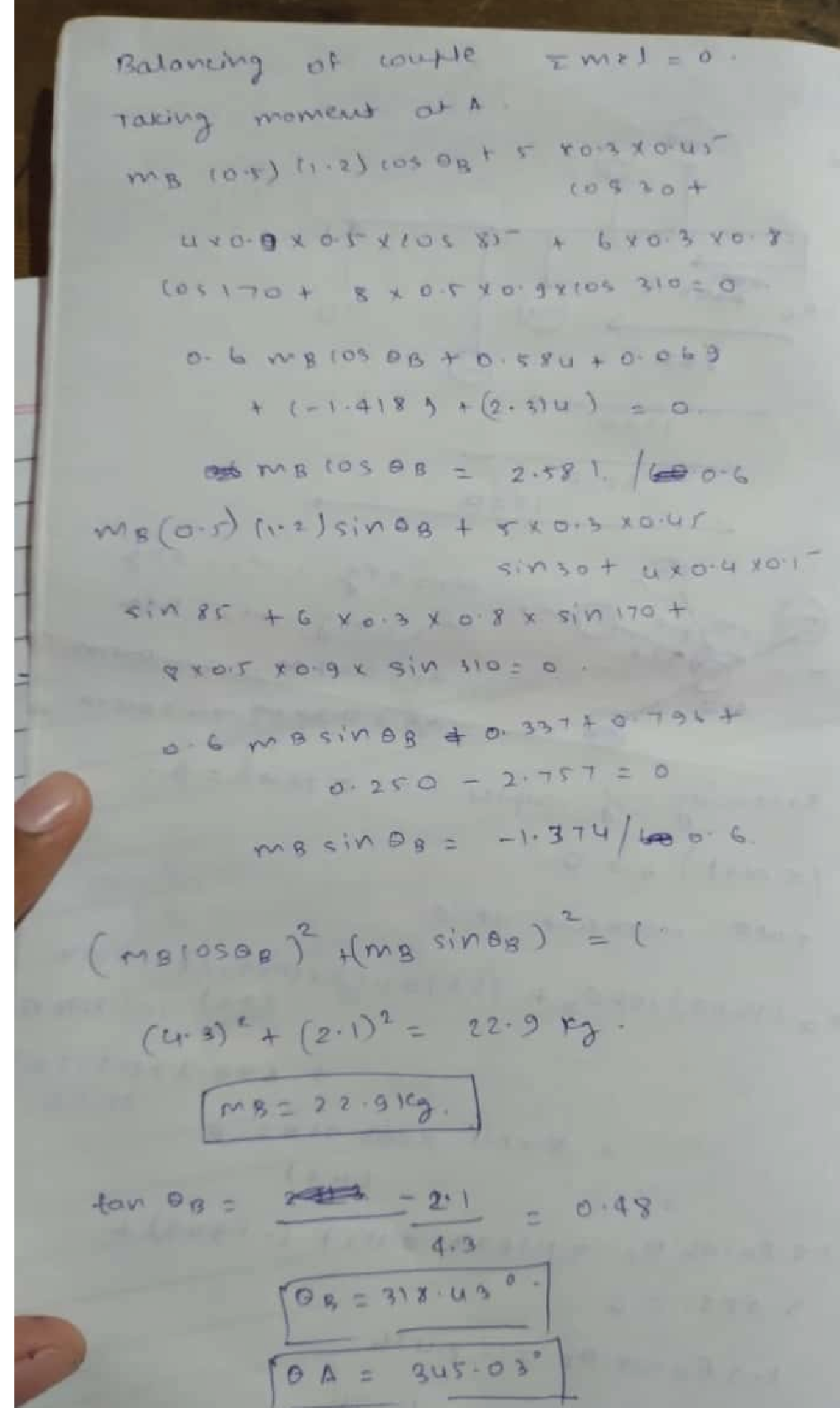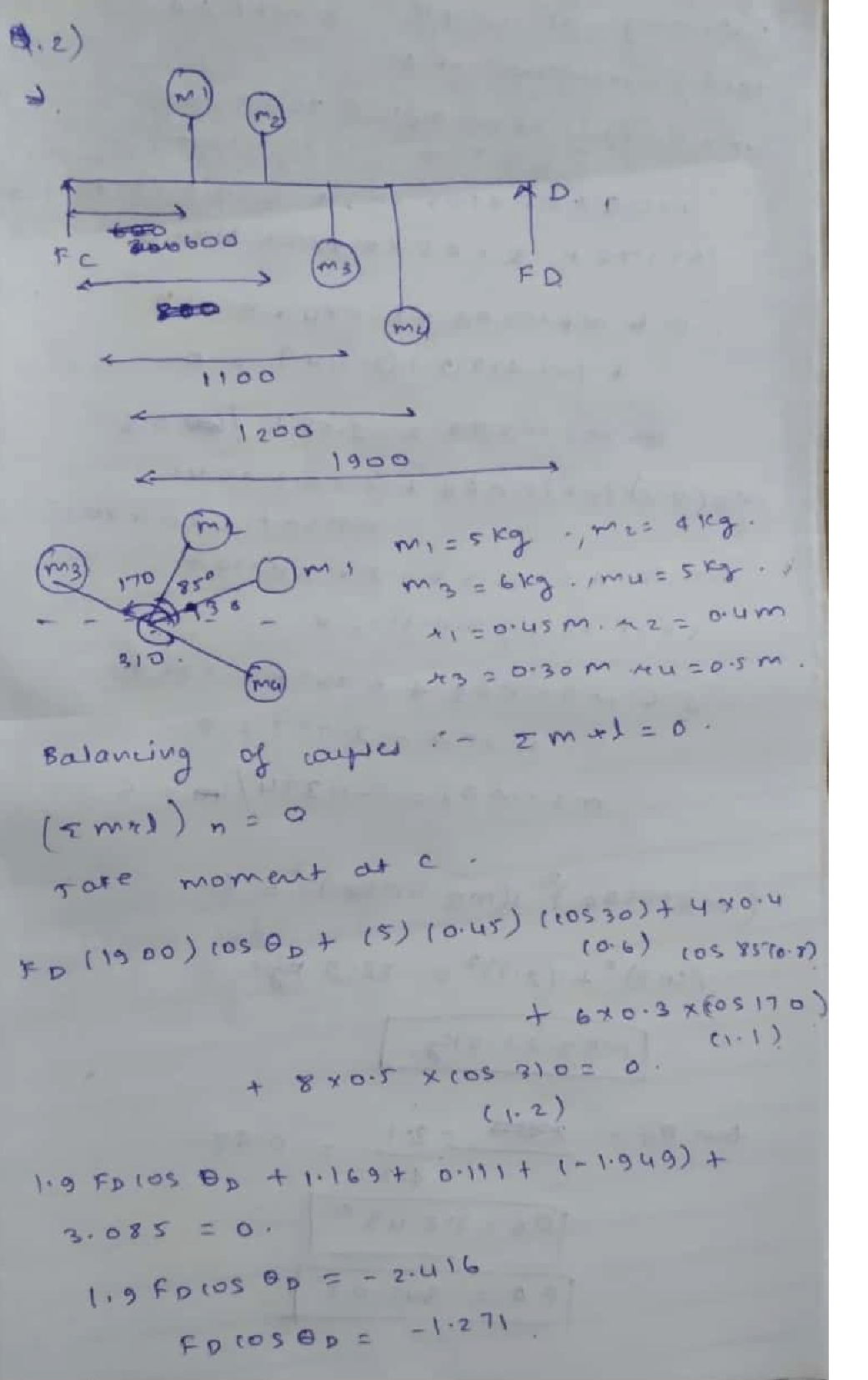Question

Machine Design

A system of four non-coplanar weights is arranged on a shaft (the angles and lengths on the figure are arbitrary), as shown in Figure 1. The shaft rotates at 200 rpm, LB =1200 (mm.) Assume the following data:

For the unbalanced System, Figure 1 (Without the Two counterweights(ma and mB, at planes A & B respectively), calculate the magnitudes and relative angular orientations of the bearing reaction at C & D, Fill your answers in the following table

Determine the masses (ma and mg) and angles of the counterweights incorrection planes A and B needed to dynamically balance the system, at RA400 mm., and Rg = 500mm. The correction planes are Lg =1200 (mm.)apart. Fill your answers in the following table:Verified### Question 45727Machine Design

a) Draw the FBD of pulley at B and pulley at A using compasses for circles
b) Use torque equilibrium, to find the tensions in the belt on pulley A assuming the shaft is running at a constant speed
c)find the magnitudes of the bearing reaction forces at O and C, assuming Draw the FBD of the shaft and the bearings act as simple supports
d) Draw shear-force, bending-moment and torque diagrams for the shaft. If needed, make one set for the horizontal plane and another set for the vertical plane.
e)Find the critical point on the shaft and, determine the bending stress and the torsional shear stress. Why is this point critical?
f)Draw the stress state for this point and show the stresses on an infinitesimal square element
g) Draw the Mohr circle on a scaled paper with rulers and compasses
h) Find the principal stresses and maximum shear stress at this point
i)Show the stress state at the principal and at maximum shear stress planes

### Question 45726Machine Design

A countershaft carrying two V-belt pulleys is shown in the figure has a diameter d=33 mm. Pulley A receives power from a motor through a belt with the belt tensions shown. The power is transmitted through the shaft and delivered to the belt on pulley B.Assume the belt tension on the loose side at B is 9 percent of the tension on the tight side. T1=1925 N, T2=385 N.
1- Find the tension T3=
2- Find the tension T4 =
3- Find the reaction at the support C in y direction, Cy,=___N
4- Find the reaction at the support O in y direction,oy=n
5- Find the reaction at the support C in z direction cz=___N
6- Find the reaction at the support O in z direction, 0z =____N
7- Find the total resultant moment at point A, MA____N.mm
8- Find the total resultant moment at point B, MB=---N.mm
9- Calculate the normal stress at point A, theta A____mpa
10- Calculate the normal stress at point b theta =mpa
11- Calculate the torque at point A, TA=_____N.mm
12- Calculate the torque at point B, TB=______N.mm
13- Calculate the shear stress at point A, TA=____MPa
14- Calculate the shear stress at pointВ, Тв-----MPа
15- Calculate the maximum principal stress, theta 1 =MPa
16- Calculate the minimum principal stress, o2 =___MPa
17- Calculate the maximum shear stress, Tmax____MPa
19- Calculate the angle to the maximum shear stress planes, theta =___ deg
18- Calculate the angle to the principal planes,theta p=__ deg

### Question 45725Machine Design

Explain the following items by writinga paragraph and drawing figures
a) Yield strength,
b) Ultimate tensile strength
c) Principal stresses,
d) Maximum shear stress
e) Mohr circle

### Question 45451Machine Design

In the figure shown, shaft OA, made of AISI 1030 hot-rolled steel, is welded to a fix support at 0 and subjected to loading by forces F1, F2, F, and the external moment Me as shown below. The shaft has a20 mm. Any possible deformation of the shaft will take place along the x-axis only, and diameter d axial stresses are neglected. Stresses are to be considered at support O.
The forces are F120 N, F230 N, and F350 N, and Me= 10 N.m.
1. Find the moment(s) and torque(s) that each force will produce on the shaft at O [4.5 Marks]
2. Find the resultant moment and resultant torque at O [4 Marks
3. Find the bending and shear stresses at O [3 Marks]
4. Find the factor of safety for shaft OA by using the Maximum Shear Stress theory and specify whether the structure is safe or not 3.5 Marks]

### Question 44978Machine Design

A full journal bearing is 29 mm long. The shaft journal has a diameter of 58 mm with a unilateral tolerance of-0.012 mm. The bushing bore has a diameter of 58.05 mm with a unilateral tolerance of 0.012 mm. The load is 2 kN, and the journal speed is 658.1786 rev/min.For the minimum clearance assembly, find the minimum oil-film thickness, the power loss, and the side flow if the operating temperature is 65°C and SAE 40 lubricating oil is used.
The minimum oil-film thickness is___mm.
The power loss is___W.
The side flow is____mm3/s.

### Question 44977Machine Design

A full journal bearing has a journal diameter of 34 mm, with a unilateral tolerance of -0.012 mm. The bushing bore has a diameter of34.05 mm and a unilateral tolerance of 0.032 mm. The bearing is 68 mm long. The journal load is 1 kN, and the journal runs at403.7907 rev/min. Using an average viscosity of 55.6 mPa-s, find the minimum film thickness, the maximum film pressure, and the total oil-flow rate for the minimum clearance assembly.
The minimum film thickness ismm.
The maximum film pressure is___Mpa.
The total oil-flow rate for the minimum clearance assembly is___mm3/s.

### Question 44265Machine Design

11-1 Timken rates its bearings for 3000 hours at 500 rev/min. Determine the catalog rating for a ball bearing running for 10,000 hours at 1800 rev/min with a load of 2.75 kN with a 90% reliability.

### Question 42609Machine Design

The input shaft of the gear box shown has a gear A with 40 teeth, which meshes with gearB on the lay shaft having 60 teeth. The lay shaft has an output gear C having 50 teeth,which meshes with gear D on the output shaft having 60 teeth. Compute:
A. The gear ratio.
B. The speed of the input shaft, if the speed of the output shaft is 1800 rpm.
C. The centre distance (L).
E. The circular pitches of A/B and C/D.1000 AL
D. The module of gears A and B, if the module of gears C and D is 4 mm.
F. If the force of compression approximately equals to 1000 N, find the torque ratio andthe efficiency on the input and output shafts.

### Question 42283Machine Design

The input shaft of the gear box shown has a gear A with 40 teeth, which meshes with gear B on the lay shaft having 60 teeth. The lay shaft has an output gear C having 50 teeth,which meshes with gear D on the output shaft having 60 teeth. Compute:
A. The gear ratio.
E. The circular pitches of A/B and C/D.
B. The speed of the input shaft, if the speed of the output shaft is 1800 rpm.
D. The module of gears A and B, if the module of gears C and D is 4 mm.
C. The centre distance (L).
F. If the force of compression approximately equals to 1000 N, find the torque ratio and the efficiency on the input and output shafts.

### Question 41387Machine Design

For the bearing application specifications given in Table A, determine the Basic Load Rating for a ball bearing with which to enter a bearing catalog of manufacturer 2 in Table 11-6. Assume an application factor of one.

### Submit query

Getting answers to your urgent problems is simple. Submit your query in the given box and get answers Instantly.

### Submit a new QuerySuccess

Assignment is successfully created